# GraphSight 2.0.1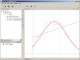GraphSight is designed for 2D math graphing needs.

 Developer: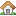Cradle Fields, Inc.      software by Cradle Fields, Inc. → Price: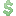29.00  buy → License: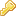Trial File size:0K Language: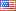OS: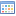Windows Vista (?) Rating: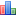0 /5 (0 votes)

GraphSight is designed for 2D math graphing needs.

GraphSight makes the process of math function plotting and analyzing a matter of a seconds. You may plot several functions in the same window, create several coordinate spaces defined by different variables (not only y,x), set labels on the plot, and use custom user-defined constants in every formula. All of the graphical objects on the plot are much interactive.

Whether you a student, an engineer or a teacher, it happens you spend much time investigating functions behavior. Usually, the derivatives of the first and second order, intervals of increasing and decreasing, inflections of the curve, roots of special equations are found and analyzed to just plot a graph. GraphSight does the entire thing for you. It plots graphs thus letting you set about real research instead of performing graphing shores.

GraphSight is a convenient graphing and curve fitting utility for Windows designed to quickly plot and explore 2D math functions and data. GraphSight easily plots common x(y), y(x) Cartesian, parametric, polar and table-defined graphs.

GraphSight curve fitting engine uses least squares method to perform regression analysis (different regression types are supported). This tiny powerful graphing software gives you a full control over a coordinate plane through the interactivity of every graphics on it

###### GraphSight features
• Plot common Y(x), X(y) Cartesian, polar, parametric and table-defined graphs.
• Carry out curve fitting routines. Regression analysis (linear, gauss, polynomial, reciprocal, hyperbolic, logarithmic fits are available) is done using least squares method.
• Trace curves. It makes it possible to visually observe how the function Y(X) depends on the variable X.
• Analyze data. Correlation coefficient, mean values and standard deviations of data vectors are calculated.
• Automatically adjust coordinate grid and axes appearance.
• Zoom or shift a graph with just a single click & move of mouse.
• Print the resulting picture or export it to a file or windows clipboard as a bitmap.
• Keep several coordinate spaces opened at a time.
• Undo and Redo changes made to graphs.

###### Requirements
• Internet Explorer 5.0 or higher installed.

###### Limitations
• 30 days trial

tags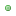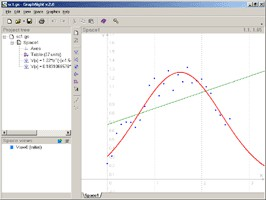# Download GraphSight 2.0.1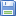Download GraphSight 2.0.1

Purchase: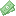Buy GraphSight 2.0.1

###### Similar softwareGraphSight 2.0.1
Cradle Fields, Inc.

GraphSight is designed for 2D math graphing needs.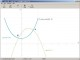GraphSight Junior 1.0
CradleFields.com

GraphSight is an application that can be used at 2D math-graphing projects GraphSight Junior is an easy to use freeware handy 2D math-graphing program.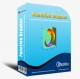Function Grapher 3.9.2
GraphNow

Function Grapher is a easy-to-use software for 2D, 2.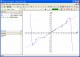Advanced Grapher 2.2
Alentum Software, Inc.

Advanced Grapher - Powerful but easy-to-use graphing, curve fitting and calculating software.ZigZag 1.3
Palam Software, Inc.

ZigZag is a handy tool to plot and explore math functions.LAB Fit Curve Fitting Software 7.2.38
Wilton Pereira da Silva

LAB Fit Curve Fitting is an application that can help with the process and analysis of experimental data.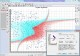FindGraph 2.611
UNIPHIZ Lab

Are you an engineer, scientist or graduate student looking for a graphing tool that will allow you to quickly analyze graphed data without having to immerse yourself into calculus and statistics books? You've just found the perfect solution.Graph Plotter 1.2.3
Still Soft

Graph Plotter is a powerful utility that will help you plot and analyze different types of graphs.Rt-Plot 2.8.10.83
Rt-Science

Rt-Plot is a useful tool that will generate Cartesian X/Y-plots from scientific data.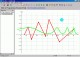ZGrapher 1.4
Palam Software Inc

ZGrapher is a useful utility with the help of which you can create and explore graphs.